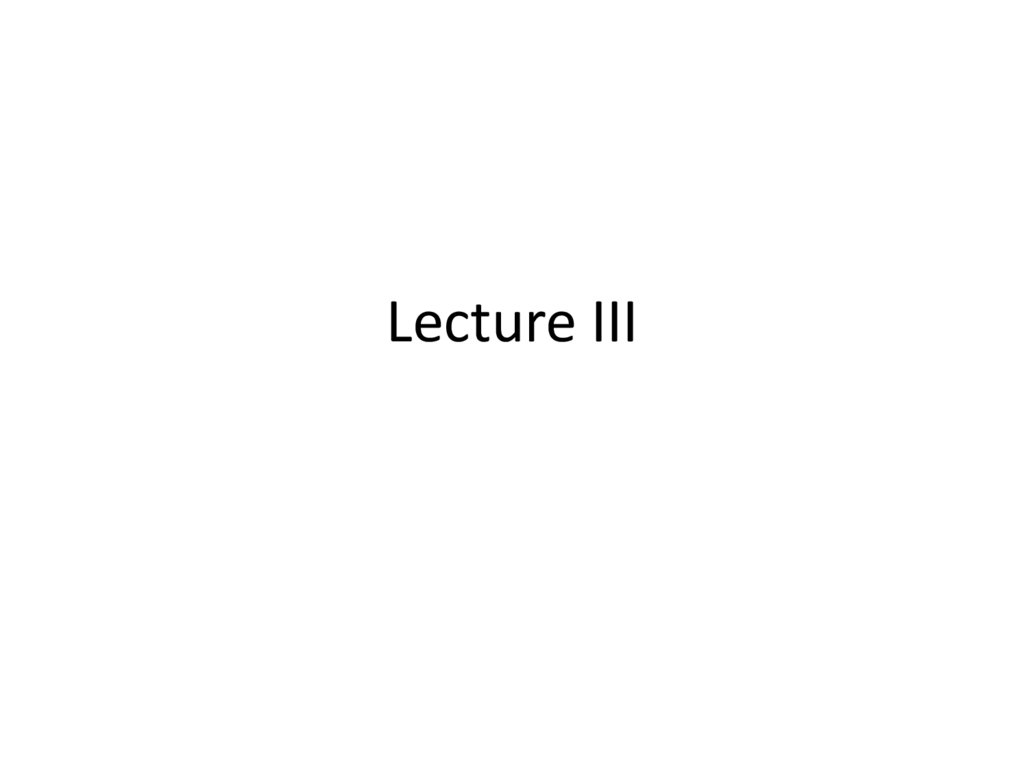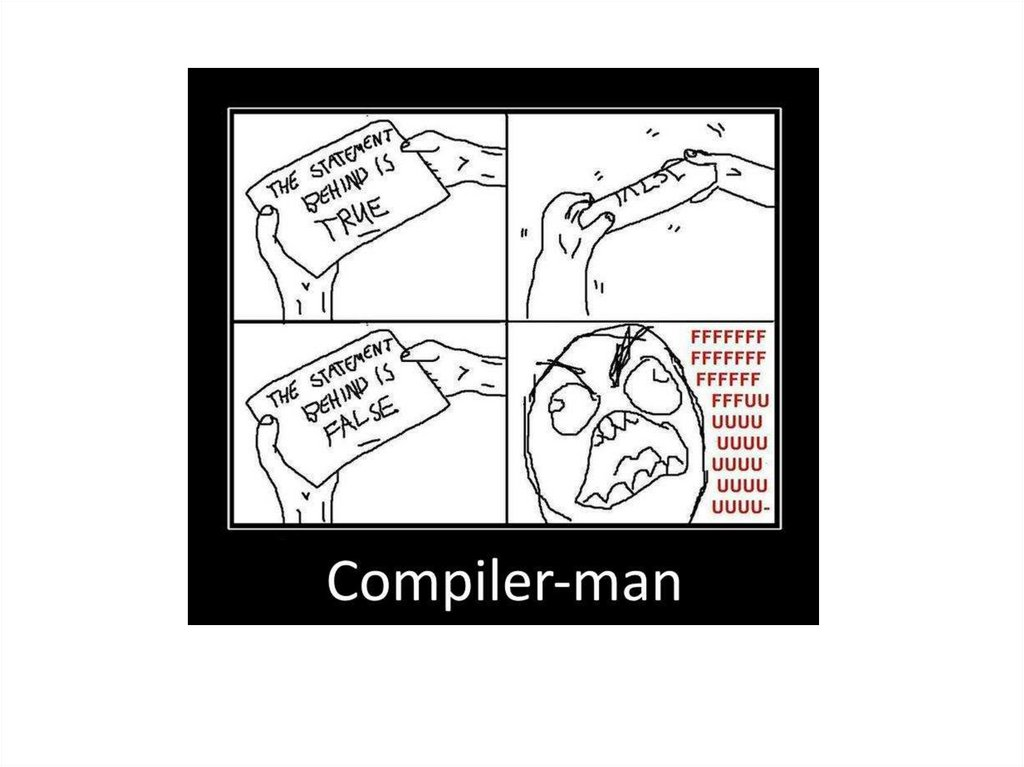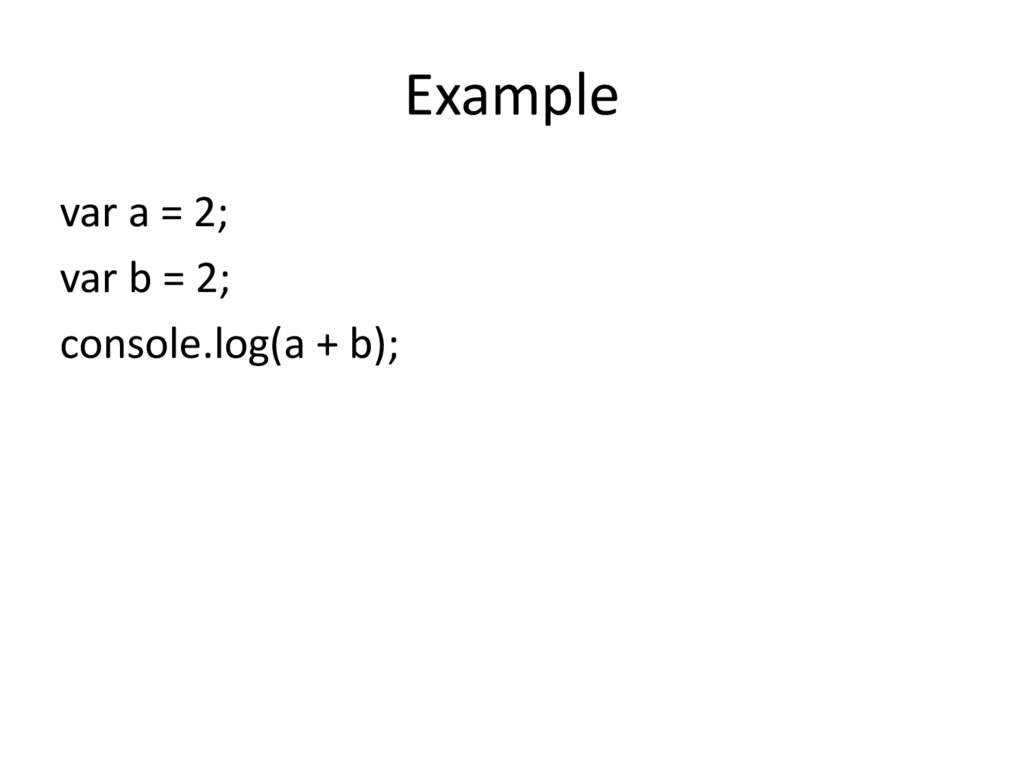# Scope in ES3 World

Lecture III

## 3.

Example
var a = 2;
var b = 2;
console.log(a + b);

## 4.

Recap of the previous lecture

## 5.

Scope in ES3 World

## 6.

The Scope of a Variable
The scope of a variable are the locations
where it is accessible.
For example:
function foo() {
var x;
}

## 7.

Scope - is a logical boundaries in which a
variable (or expression) has its meaning.
For example, a global variable, a local
variable, etc, which generally reflects a logical

## 8.

Scope can be nested
function f() {
var x = 2;
function g() {
var y = 3;
}
}

Nested Scope
outer scope
function f() {
var x = 2;
function g() {
var y = 3;
}
}
inner scope

## 10.

var scope = "global ";
// A global variable
function outer() {
var scope = “outer”;
// A outer variable
function inner() {
var scope = “inner";
document.write(scope);
// Prints "inner"
}
inner();
}
outer();

## 11.

var scope = "global ";
// A global variable
function outer() {
var scope = “outer”;
// A outer variable
function inner() {
document.write(scope);
// Prints "outer"
}
inner();
}
outer();

## 12.

var scope = "global ";
// A global variable
function outer() {
function inner() {
document.write(scope);
// Prints "global"
}
inner();
}
outer();

## 13.

function X() {
var a = 3, b = 5;
function foo () {
var b = 7, c = 11;
a += b + c;
};
foo();
alert("a = " + a + "; b = " + b);
}
X();
var x = function(){
}
x();

## 14.

Hoisting
JavaScript hoists all variable declarations, it
moves them to the beginning of their direct
scopes.

## 15.

Hoisting
foo(); // undefined ("foo"
and "bar" exist)
function foo() {
}
function foo() {
}
var bar;
var bar;
foo(); // undefined

## 16.

Hoisting
var scope = "global ";
function f( ) {
var scope = "local";
}
f( );
var scope = "global ";
function f( ) {
var scope;
scope = "local";
}
f( );

## 17.

Only functions introduce new scopes
No block scope
function f() {
{ // block starts
var foo = 4;
} // block ends
console.log(foo); // 4
}

## 18.

No block scope!
function test(o) {
var i = 0;
// i is defined throughout function
if (typeof o == "object") {
var j = 0; // j is defined everywhere, not just block
for(var k=0; k < 10; k++) { // k is defined everywhere, not just loop
console.log (k);
}
console.log (k); // k is still defined: prints 10
}
console.log (j); // j is defined, but may not be initialized
}

Hoisting
var foo = 1;
function bar() {
if (!foo) {
var foo = 10;
}
}
bar();

## 20.

Code in global scope
Index.html
<!DOCTYPE html>
<html>
<title>Untitled Page</title>
<script type="text/javascript" src="script.js"></script>
<body>
<script type="text/javascript">
script.js
var a = 5;
var b = 2;
var gloabalVar = 5;
function sum(x, y) {
return x + y;
}
function mul(x, y) {
return x * y;
}
</script>
</body>
</html>
function square(x) {
return x * x;
}

## 21.

Global namespace
Every variable is global unless it's in a function and
is declared with var
function f(){
x = “global variable”;
}
f();

## 22.

Global object (WAT)
function f(){
x = “global variable”; // var is missed
}
f();
this.x === “global variable”;
window.x === “global variable”; // true for browsers

## 23.

window vs global
(function (glob) {
// glob points to global object
}(typeof window !== 'undefined' ? window : global));

## 24.

Global variables are evil
• They are less robust, behave less predictably,
and are less reusable.
• Name clashes. Your code, built-ins, analytics
code, social media buttons use the same
global scope.

## 25.

Globals
// antipattern
function sum(x, y) {
// implied global
result = x + y;
return result;
}
// antipattern
function foo() {
var a = b = 0;
// ...
}
// preferred
function foo() {
var a, b;
// ...
a = b = 0; // both local
}

## 26.

<script>
if (("a" in window) == false) {
var a = 1;
}
</script>

## 27.

Working with global
window.foo
if (window.foo) { … }
if (“foo” in window) { … }
if (typeof “foo” !== “undefined”) { … }

## 28.

Namespaces
if (window.myNamespace == null){
window.myNamespace = {};
}
window.myNamespace.myFunction = function(/* params*/ ) {
/* code here */
};

## 29.

immediately invoked function
expression and ES6 Modules
in next lectures

## 30.

REFERENCES
JavaScript: The Definitive Guide, Six Edition
by David Flanagan
Speaking JavaScript: An In-Depth Guide for Programmers
by Dr. Axel Rauschmayer
http://dmitrysoshnikov.com
http://learn.javascript.ru